# Optimize the Energy Consumption of your Electronic DeviceEnergy consumption is an important parameter that not only concerns sustainability. It is true that by optimizing the use of energy on the device, less energy will be required to perform its function, therefore more efficient, but also by performing this optimization you will have an overall better product.

For example, if the product is battery powered then you can extend its battery life or even better reduce the size of the battery, lowering costs and reducing the embodied energy of the product. Everybody wins.

As with many things in life, optimizing power consumption in an electronic device follows the Pareto principle or 80-20 rule. Around 20% of the modifications will produce 80% of the results (energy wise, in Wh for this case).

A distinction should be made between a battery and non-battery powered systems. Some of these optimizations can drastically reduce the energy consumption of the device, such optimizations should be then applied to both battery and non-battery powered devices.

On the other hand, some optimizations will only improve the energy consumption of the system by a few mA’s. It only makes sense to apply these optimizations for battery-powered systems, where that small fraction of current is actually relevant.

At the end of the article, a comparison will be made between an optimized and non-optimized system hence the impact of the changes can be visualized.

## MCU-level optimizations

In many embedded systems, the MCU will be one of the main culprits of high power consumption if left unchecked. MCU power consumption can be divided into two states: dynamic and static.

### Dynamic Power

Dynamic power consumption is the current consumed during the normal operation of the MCU. It includes the power lost in switching the internal CMOS transistors that normally control functions such as the digital output and other MCU circuits like the ADC modules and the clock oscillator.

The dynamic power consumption can be defined by the following equation:

P = V^2*f*C

Where V is the voltage supplied to the MCU, f the frequency of the CMOS gate and C the CMOS capacitance and external capacitance.

Consider the following typical push-pull digital output diagram from an MCU pin: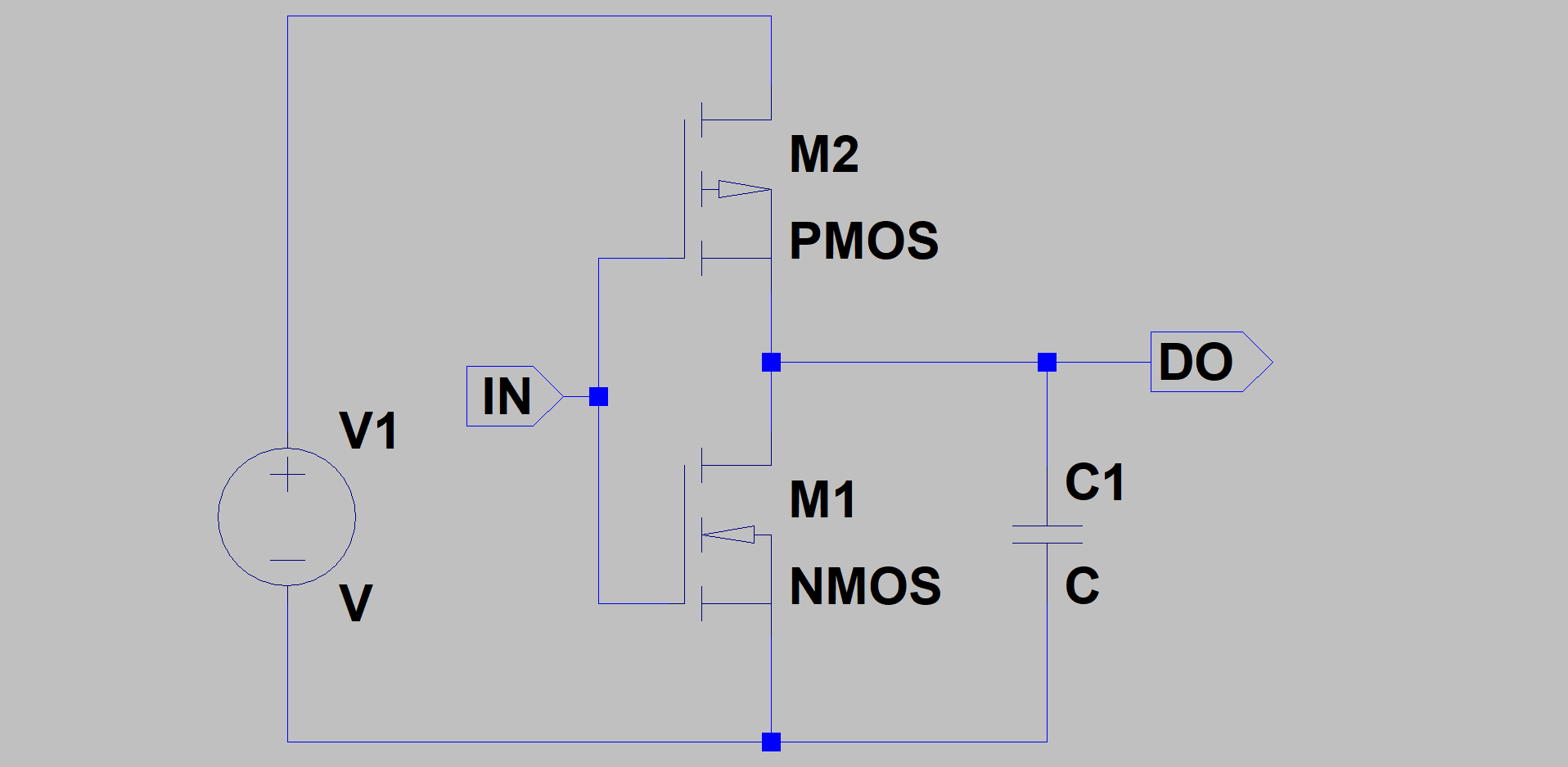If we see it from a static point of view, when DO is Vcc or GND, there is virtually no power consumption as no current is flowing (this doesn’t take into account what is connected to DO).

However, if we analyse it dynamically, during the transitional states from Vcc to GND and vice-versa, the capacitor charges and discharges, hence power is wasted.

By looking at the previous equation, we can see that the most important variable regarding consumption is the MCU is voltage. For that reason, it is a better option to choose a lower voltage MCU. Most modern MCU’s run at 3.3V while some of them at even lower voltages such as 1.2V. If not required by your application, try to avoid powering your MCU with 5V.

Another parameter is the external capacitance.  It is better to avoid high C values at the MCU DO’s. Anyhow, if you have a high-speed signal connected to that pin such as an SPI clock, you cannot put a big capacitor as you will over damp the signal (make the square wave a curvy squared wave) and the communication between the devices will not work.

Regarding the frequency, initially, it seems that by having a lower switching frequency there is less power consumption. However, you must fact into account that by having a lower switching frequency the component will also take longer to finish the task, therefore consuming current from another part. As we will see later, it is more efficient to finish quickly the task and then enter into low power mode.

### Static Power

Static power is the power consumed by the MCU while this is not actively running code, therefore in sleep or low power mode. The current consumed in this state is drawn mainly by quiescent currents from the internal voltage regulator, BOR circuit and internal oscillators. Also, some current is lost from leakage.

### Clock frequency

What will consume more current? setting the clock at 2 MHz or 16 MHz?

Running the clock itself will consume more current. However, it turns out that the initial current required to power the oscillator represents a significant portion of the total power consumption at low frequencies, on the other hand, at high frequencies it becomes negligible.

The result is that it is more efficient to run the code at higher frequencies since its uA/MHz value will be lower.

Bear in mind that for this principle to apply, the oscillator must be powered by a low impedance voltage source that can quickly deliver the required current

### Peripherals

Selecting and configuring properly the MCU peripherals can help to reduce power consumption.

#### High vs. low-speed peripheral clocks

When choosing the clock speed of peripherals there are two variables to take into account: the current consumption of the peripheral and the time it takes to perform its required task.

Some serial communications protocols such as UART will tend to use less current than SPI or I2C, however, a UART takes more time to send a Byte compared to other protocols, making  the total charge higher as you can see from the following table taken from Microchip AN1416 App Note:Therefore, it is more beneficial to use a high current peripheral that takes less time to perform the task.

Internal circuits of the ADC are mainly analogue components that do not vary its consumption with speed.

Because most of the current consumption of the ADC comes from powering these components, it is more beneficial to have a high-speed conversion and then disabling the module.

#### Brown-Out Reset circuit

This MCU component monitors the supply voltage and resets the MCU if the voltage falls below a set threshold.

This circuit can consume a considerable amount of current while in sleep or low power mode. For that reason, it is better to turn it off while the MCU enters into this state. Since the MCU is not executing code, the BOR protection is not really necessary.

#### Unused pins

Unused pins that are left floating, can couple with an external signal and produce a random voltage which can bias the internal transistors and cause a current consumption of up to 100uA.

To avoid this situation, configure any unused pin as a digital output and drive it to either low or high

## Power Supply Decisions and Design

As you can imagine, choosing the correct type of power supply to have a central role in optimizing the energy efficiency of the device. Apart from the cons and pros of each topology, other parameters such as how much current the supply needs to operate and its efficiency need to be carefully analysed to choose the most optimal solution.

### Use cases Linear vs Switching

It is well known that switching power supplies are much more efficient than linear regulators. This is because losses in SMPS are mainly a factor of the internal resistance and switching frequency of the MOSFET used plus the voltage drop at the diode, while in linear regulators most losses come from the voltage drop at the regulator transistor.

To illustrate the difference, let’s do a calculation to compare the power losses assuming a voltage conversion from 9V to 5V at 1A of current (note that this calculation does not take into account quiescent current):

For a linear voltage regulator:

P_loss = Vin-Vout*Iout = (9V-5V)*1A = 4W

For a step down converter (assuming a Fsw of 2Mhz, transient time of 40ns and RdsON of 0.1ohm):

P_loss = PconMOS + PswMOS + PswD

P_loss = (Iout^2*RdsON*Vout/Vin)+(0.5*Vin*Iout*tsw*Fsw)+(Iout*Vf*(1-Vout/Vin))

P_loss = (1A*1A*0.1ohm*5V/9V)+(0.5*5V*40ns*2MHz)+(1A*0.4V*(1-5V/9V))

P_loss = 0.055W + 0.2W + 0.178W

P_loss = 0.433W

As you can see, an SMPS can have a 10 times less power loss compared to a linear regulator.

However, a linear regulator can be a better option in some situations.

SMPS is an ideal choice when the difference between Vin and Vout is more than a couple of volts and a high current constant load needs to be powered, as they provide much greater efficiency as shown in the calculations.

But when a device spends most of its life in sleep mode, consuming below 1mA of current, a linear voltage regulator should be considered. As you can see from the following table taken from the LM2621 datasheet, SMPS become less efficient at low currents: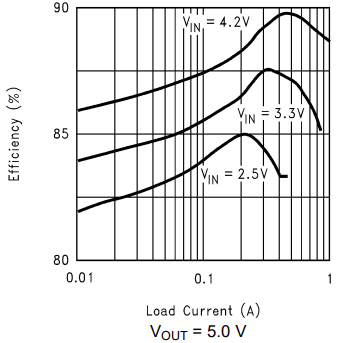Therefore, for embedded systems with long sleep times, a linear voltage regulator should be considered as a viable a choice.

### Voltage Regulator Efficiency

Efficiency is understood as the ratio of the power lost in the conversion process given by the equation:

Pout = Pin*eff

The efficiency is determined by the power losses as seen above plus the power required for the component to work

### Quiescent current

Another parameter to watch out for is the required current for the voltage regulator to do the voltage conversion, or also known as quiescent current.

This parameter can easily be found on the component’s datasheet (7805A and LM2621):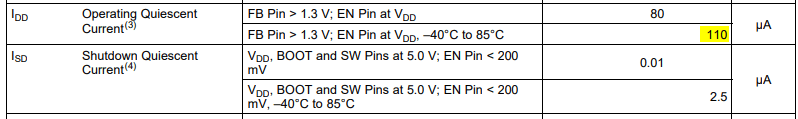### High Efficiency Switching Regulators

Silicone manufacturing technology and design techniques improve every year. This allows IC manufacturers to create better components in many aspects, such as improving their efficiency.

Here is a comparison between two step up converters efficiencies, the LM2621 (released on 2004) and the TPS61230 (released on 2014):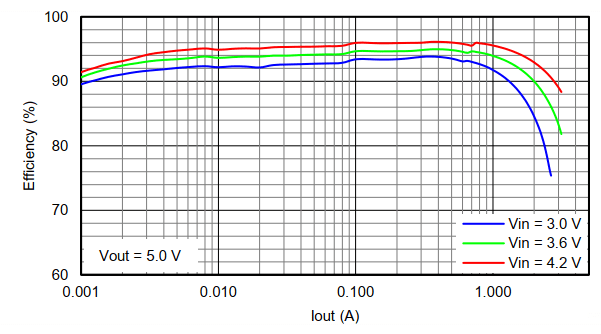As you can see, the newer device has a better efficiency, particularly at low currents.

Pricewise, the LM2621 costs around 2.30 USD and the TPS61230 2.90 USD. Therefore, the extra cost could be justified if the 10% gain in efficiency saves energy in the long run.

### High Efficiency Linear Regulators

Linear regulators need to drop a minimum amount of voltage at their input in order to supply a certain amount of current.

Here is a comparison of the required voltage drop between the classic 7805A (very old) and the TPS748 (released in 2007):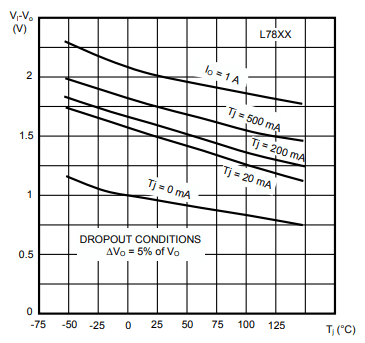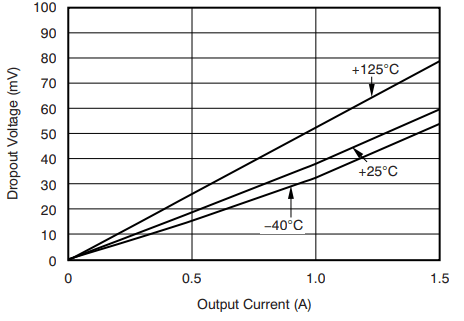As you can see from the above graphs, the 7805A needs to drop at least 2V in order to source 1A of current at 25C while the TPS748 only needs to drop 40mV to source the same current at the same temperature. This is a massive improvement.

The price of the 7805A is 0.42 USD while the TPS748 is 2.14 USD. As in efficiency improvement, there is also a massive difference in price.## Components and ICs

This section dives into low-power design techniques for Integrated Circuits and passive components.

### IC’s Power Management

The total current consumption of a device, as seen in a powertree analysis, is mostly made by the sum of all IC’s and  MCU current.

So, in order to reduce current consumption, it makes sense to turn off as many IC’s as we can while they are not being used.

One quick and convenient way to achieve this is by using the EN pins that you can find in most current demanding IC’s. When in sleep/disabled mode, the IC will normally shift from consuming mA’s to uA’s.

It is good practice to always buy IC’s with a low quiescent current. This is normally advertised on the first page of the datasheet.

In AC mains powered systems, this solution is normally more than enough, however in battery-powered systems with very tight power budgets, sometimes you need to disconnect the device from the power rail in order to remove all current consumption.

This can easily be achieved by using a transistor as a switch controlled by the MCU.

### High side or Low side switch?

Or in other words, is the transistor connected from Vcc to the IC or from the IC to GND?

Power wise, the difference is negligible, so the choice comes down to the following design parameters:

 Safety Signal integrity Cost/Complexity High side In the automotive industry, it is considered safer to use a high side switch as the load will be always connected to GND (chassis) No disturbances on the reference GND Uses two transistors and three resistors Low side – Having an element between the load and GND will create a small voltage shift that will offset the reference GND of that particular IC. This can create problems when interfacing the IC with other circuits. If the current is very low or the transistor’s RdsON/Vce is small, then this shouldn’t be an issue Uses one transistor and two resistors

#### BJT or MOSFET?

Bipolar Junction Transistors require a base current which needs to go through the MCU if the transistor is connected to one of the digital outputs. Since MOSFETs require virtually no input current at the gate, MOSFETs are a better choice if the objective is to use less power.

Also, when calculating power dissipation, a MOSFET will tend to dissipate less. For a 10mA load over a cheap MOSFET with RdsON of 4 ohms, we have a dissipation of:

Pdis = 0.01A*0.01A*4ohm = 400uW

While for a BJT driving the same current and a voltage drop between collector and emitter of a typical of 50mV we have a dissipation of:

Pdis = 0.05V*0.01A = 500uW

#### Example High side circuit

The following circuit can be used to turn ON/OFF different IC’s with low currents (around 10mA) and small bypass capacitance (around 100nF). R2 is not essential, however, it is good practice to place a pull-down resistor in order to guarantee a low level in case that the IN pin is floating or high impedance.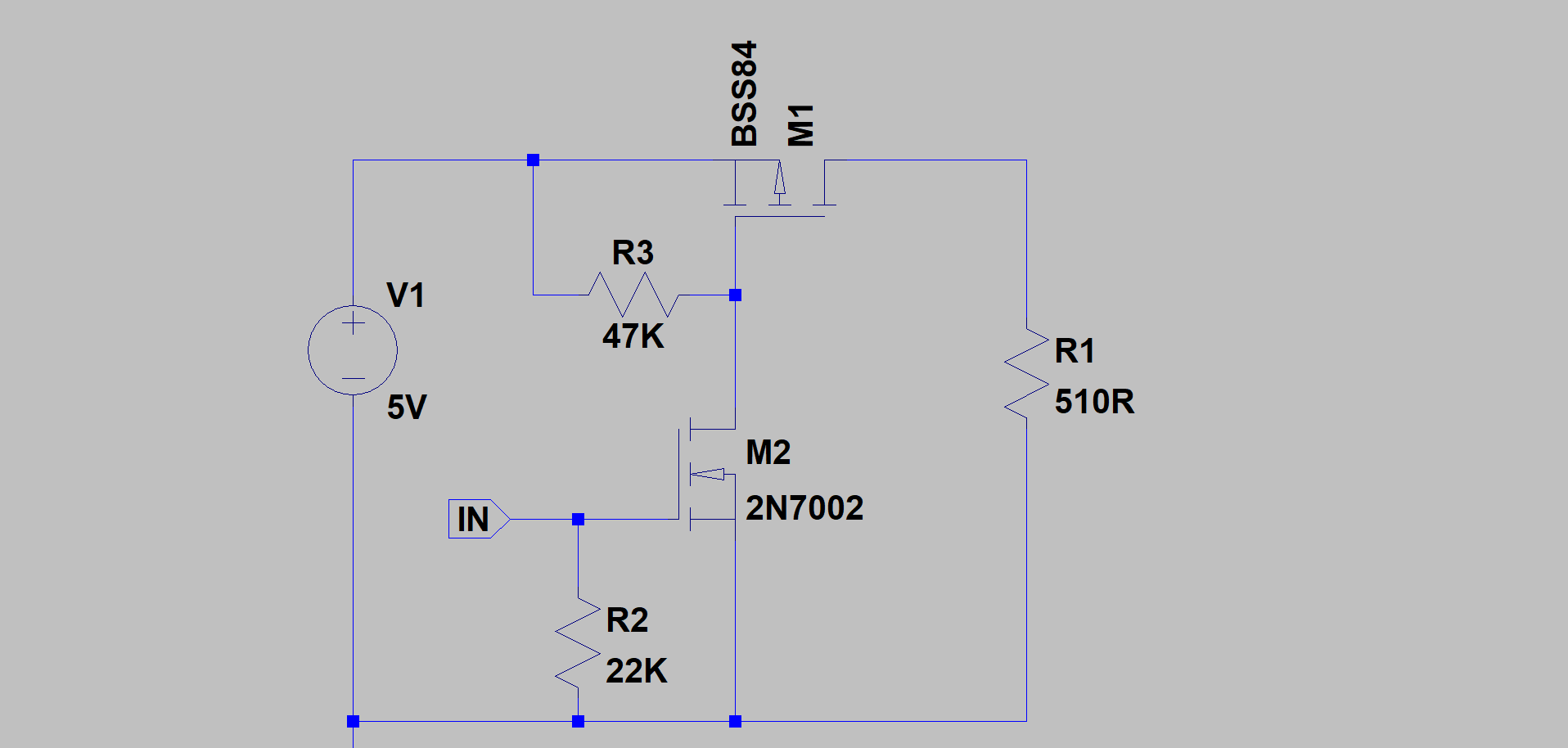### Capacitors Leakage Current

After fully charging a capacitor, a tiny amount of current will still be flowing between the positive and negative plates. This is defined as the capacitor’s leakage current.

Leakage current is a function of the dielectric used, the voltage applied to the capacitor and the capacitance.

Normally, electrolytic capacitors will have a leakage of uA’s while ceramic capacitors in the order of nA’s.

For most of your IC’s bypassing, you will probably use ceramic capacitors with 10uF or less capacitance. In this case, leakage is not really a problem in terms of power consumption.

However, in a battery system that requires big electrolytic capacitors for decoupling or bypassing, leakage can become an issue.

As a general rule of thumb, avoid the use of high capacitance electrolytic capacitors in battery powered systems.

### Pull-up/down resistors

Resistors hanging from the power supply line with a path to GND are a constant source of current consumption on the circuit.

As a general rule, when a pull-up resistor is needed by any circuit, you should try to use the highest value possible, which is typically 47K or 51K ohms.

This works great for signals that are not linked to a communication bus. For pull-ups connected to high-speed communication lines such as I2C or SPI buses, a high value will deform the shape of the waveform, therefore these need to be “tuned” taking into account the speed of the bus and the stray capacitance of the line.

The resistors sizing guideline also applies to voltage dividers. Instead of using a 1.2Kohm with 3.3Kohm, try using a 12kohm with a 33Kohm resistor. Your circuit will be less immune to noise, but depending on the application, this could be acceptable.

### PCB copper traces

Where possible, on power traces that will handle a considerable amount of current, make the PCB copper traces as wide as possible.

You can also pour all the signal path with copper as it was done for the Solar Mobile Charger and Powerbank PCB. This technique will reduce the resistance of the track, therefore less heated will be dissipated.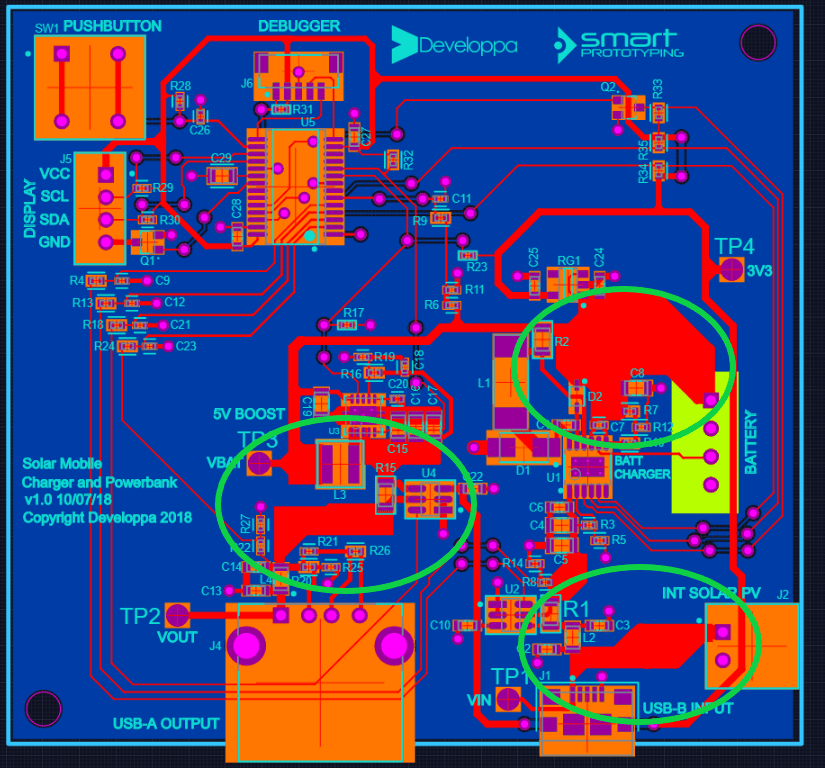## Software-based techniques

At the beginning of the article, it was mentioned that some of the optimizations explained have a very high impact on the power consumption of the device, while other will just improve it a small amount.

Software optimization is probably the most relevant tweak you can do to your device in order to reduce its power consumption.

### Interrupt based SW

Interruptions are events triggered by either an external signal or an internal peripheral of the MCU such as a timer or ADC conversion flag.

An interrupt based software operates on the premise that it only needs to execute code when certain inputs or conditions are present, the rest of the time the circuit goes to sleep.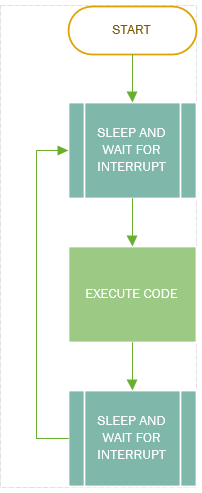Most embedded systems require a sort of input/condition such as the press of a button, elapsed time or any other external events.

Code should always be optimized and designed so during these “waiting times”, the MCU goes into low power mode instead of being in a while loop waiting for a condition to happen.

Not all waiting times are the same. Sometimes you are waiting for an ADC conversion to finish which could take us, other times waiting for a button to be pressed which could take hours and in some situations, only a single peripheral of the MCU such as a PWM needs to be used.

Because of this situation, MCU manufacturers have created different low power modes. For example, on the PIC MCU family, the following low power states exist: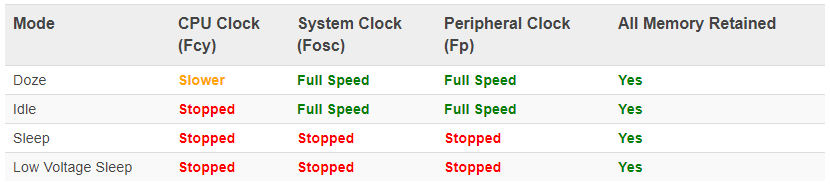Considering our previous situations we could say that each of them could be paired with a low power mode:

Waiting for an ADC conversion to finish –> go into idle state

Waiting for an external interrupt that can take more than 1ms –> go into sleep mode

Performing a single action that does not require full MCU capabilities –> go into doze mode

Implementing an interrupt based code with low power modes, can drastically improve the power consumption of a device and make a better product altogether.

### Code Optimization

Each code is different and unique, however, some effort should be put into creating ways to avoid repetitive CPU intensive tasks.

For example, in the Solar Mobile Charger, a subroutine will calculate the input power of the device by multiplying the sensed input voltage with the sensed input current. This multiplication will use floating point arithmetic, therefore it will be a CPU intensive operation.

There will be times when the input voltage and current will remain the same such as during a sunny day and while the voltage of the battery is below its nominal level.

It does not provide much useful information to know if the input power is 5.21W or 5.26W.

A previous condition before the power is calculated can be implemented where the input voltage and current are compared with the last calculation and if the measurements haven’t changed enough to affect the final result, then, skip the calculation.

Depending on the device, this strategy could make more or less sense, but it could drastically lower the power consumption on a system where the parameters vary very little.

## Optimized vs non-optimized circuit comparison

To illustrate some of the points discussed above, a fictitious device will be created.

This is an industrial device that needs to monitor and store the voltage value of 2 LiOn batteries connected in series. The device takes 10 samples of the voltage every second and then sends the data via UART to another device and stores the value on an embedded EEPROM.

The early circuit schematic of the device looks like this: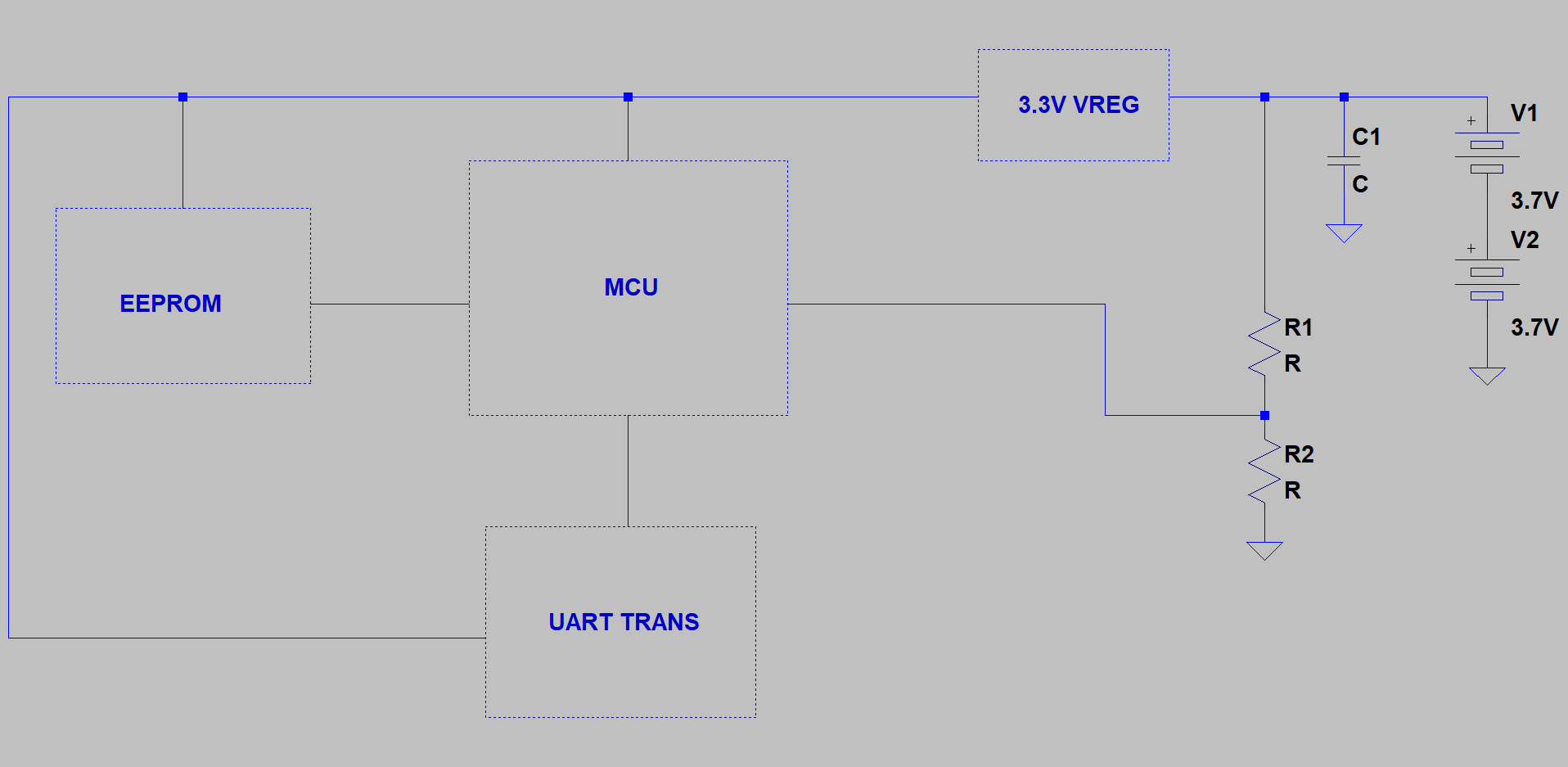A series of design decisions need to be taken:

• Value of bulk capacitance
• Value of voltage divider
• The topology of the voltage regulator
• The interface between MCU and UART transceiver
• The interface between MCU and EEPROM
• SW wakeup strategy

### Non-optimized device design decisions and max power consumption

#### Bulk capacitance

The device is far away from the batteries, therefore a big capacitor is used to keep the voltage stable and handle current peaks. A 1000uF/35V electrolytic capacitor is chosen.

The leakage current is calculated using the information provided in the datasheet:

Ileak_cap_max = 0.01*100uF*35V

Ileak_cap_max = 35uA

Ileak_cap_max = 35uA

#### Voltage Divider

For a Vin_max of 8V and a Vadc_max of 3V, a voltage divider of 5.6Kohm and 3.3Kohm is used with a current consumption of:

I_vdiv = 8/(5600+3300) = 900uA

#### Interface between MCU and UART transceiver

The TJA1044 is selected using the RX/TX lines of the MCU. The standby pin is not used, therefore the device is always on with a typical consumption of 45mA while sending data and 5mA while not sending data.

#### Interface between MCU and EEPROM

The CAT24M01 is selected. This memory communicates using I2C. The typical power consumption is 4mA when data is being written on the memory and 2uA when no operating.

#### SW wakeup strategy

This device will not go into sleep mode. After performing a conversion, sending and storing the data, it will enter a while loop until a timer of 1 second has expired and then repeat.

The PIC24FJ256GA702 is selected as the device’s MCU. The typical operating current for an oscillator frequency of 8 MHz and a Vdd of 3.3V is 2mA

#### Voltage Regulator

Before selecting the power regulator, the maximum current consumption needs to be calculated.

Note that for this example, we will use the “typical” values stated before. For a real device, the max values from the datasheet should be used so you can calculate for the Worst Case consumption.

Iout_Vreg_max = I_TJA + I_EEPROM + I_I2C + I_UART + I_MCU

Iout_Vreg_max  = 45mA + 4mA + 1mA +  0.2mA + 2mA

Iout_Vreg_max = 52.2mA

A 3.3V Linear Voltage Regulator is selected as it is the cheapest option. The max power dissipated on the voltage regulator is:

Pdiss_LDO_max = (7.4-3.3)V*0.052A = 0.213W

Now we calculate the minimum power consumption and dissipation while the device is waiting for the timer to expire:

Iout_Vreg_min = I_TJA + I_EEPROM + I_MCU

Iout_Vreg_min = 5mA + 0.002mA + 2mA

Iout_Vreg_min = 7mA

Pdiss_LDO_min = (7.4-3.3)V*0.007A = 0.028W

### Optimized device design decisions and max power consumption

#### Bulk Capacitance

The device is placed very close to the batteries, therefore the bulk capacitance required is not very high. A 22uF ceramic capacitor is selected.

The leakage is calculated using the following equation:

Ileak_cap_max= = V/(IR/C)

Ileak_cap_max = 10V/(100Mohm*uF/22uF)

Ileak_cap_max = 2.2uA

#### Voltage Divider

For a Vin_max of 8V and a Vadc_max of 3V, a voltage divider of 56Kohm and 33Kohm is used and the current consumption is:

I_vdiv = 8/(56000+33000) = 90uA

#### Interface between MCU and UART transceiver

The TJA1044 is selected using the RX/TX lines of the MCU. The standby pin is used, therefore the device can be put to sleep while not in use. The typical consumption is 45mA while sending data, 5mA while not sending data and 10uA when in sleep mode.

#### Interface between MCU and EEPROM

The M95M01 is selected. This memory communicates using SPI. The typical power consumption is 4mA when data is being written on the memory and 2uA when no operating.

#### SW wakeup strategy

This device will go into sleep mode. After performing a conversion, sending and storing the data, it will enter into sleep mode until an interrupt timer of 1 second has expired and wakes up the device.

The PIC24FJ256GA702 is selected as the device’s MCU. The typical operating current for an oscillator frequency of 8 MHz and a Vdd of 3.3V is 2mA while the typical sleep current is 4.4uA.

#### Voltage Regulator

For the optimized device, the max current consumption is virtually the same as in the non-optimized device.

Iout_Vreg_max = I_TJA + I_EEPROM + I_SPI + I_UART + I_MCU

Iout_Vreg_max  = 45mA + 4mA + 0.7mA +  0.2mA + 2mA

Iout_Vreg_max = 51.9mA

The TPS62745, an ultra-low power buck converter is selected as the voltage regulator. Using the provided efficiency graph, the power loss is calculated: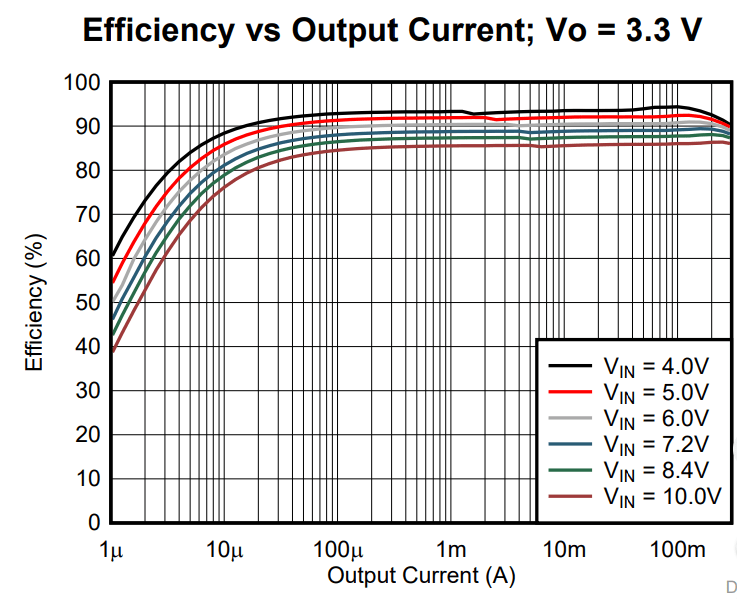Assuming an efficiency of 87% at 52mA

Ploss_buck_min= 7.4V*0.052A*0.13 = 0.05W

Now we calculate the power consumption and loss while the device is in sleep mode:

Iout_Vreg_min = I_TJA + I_EEPROM + I_MCU

Iout_Vreg_min = 10uA + 2uA + 4.4uA

Iout_Vreg_min = 16.4uA

Assuming an efficiency of 82% at 16.4uA

Ploss_buck_min= 7.4V*0.0000164*0.18 = 22uW

### Energy Analysis

To calculate the energy in Wh, firstly, two different profiles for current consumption must be calculated, then, the average current consumption can be calculated as described in the following graph from Microchip AN1416: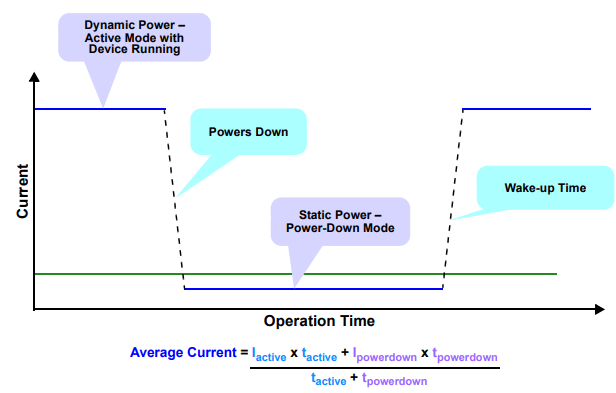#### Average current consumption

The dynamic power consumption of the device will vary depending on what part of the code the MCU is. For ease of calculation in this example, and because it is enough to illustrate the point, we will assume that the dynamic power is constant.

From our previous calculations, we have a dynamic current of 52mA for both devices.

Assuming an ADC conversion speed of 500 Ksps, 10 samples gathered and 10 bytes sent to the UART transceiver and EEPROM, the dynamic time (tactive) will be:

tactive_non-opt = (0.02+0.25+1.74) = 2.01ms

tactive_opt = (0.02+0.02+1.74) = 1.78ms

Then, the average current output of the voltage regulator is:

Iavg_non-opt = (0.052A*0.002s)+(0.007A*1s)/(1+0.002)s

Iavg_non-opt = 7mA

Iavg_opt = (0.052A*0.00178s)+(0.0000164A*1s)/(1+0.00178)s

Iavg_opt = 109uA

Both the capacitor and voltage divider before the voltage regulator are constant loads, therefore they can be directly added to our previous calculations. However, the input current of the buck converter needs to be calculated first using the following equation:

Iin_avg_buck = Iavg_opt*Vout/avg_eff*Vin

Iin_avg_buck = (109uA)*3.3V/0.845*7.4V

Iin_avg_buck = 57.52uA

Iin_opt = 57.52uA+90uA+2.2uA

Iin_opt = 150uA

Iin_non-opt = 7mA+0.035mA+0.9mA

Iin_non-opt = 8mA

#### Final Energy Consumption

The final energy consumption can now be calculated in Wh:

E_non-opt = (0.008A)*7.4V*1h

E_non-opt = 59.2mWh

E_opt = (0.00015A)*7.4V*1h

E_opt = 1.1mWh

The optimized solution will consume 53.8 times less energy than the non-optimized solution.

If we put these values in context, assuming that the batteries from our fictional device have a capacity of 10Ah, and we ignore self-discharge, then:

life_non-opt = 10Ah/0.008A = 1250h

life_opt = 10Ah/0.00015A = 66,666.7h

Going back to the initial argument of reducing the consumption so a smaller battery can be selected, thus reducing the cost of the product and its embodied energy, we could say that the optimized device could last the same amount of hours (1250h) as the non-optimized device but with a battery of:

Batt_opt = 0.00015A*1250h = 0.1875Ah

## Conclusion

In this article, we have learnt different concepts and design techniques that can reduce the power consumption of an electronic circuit.

Some of these techniques such as optimizing the software and choosing the correct voltage regulator can have an enormous impact on the final energy consumption of the device, while other guidelines such as selecting high-value pull-up resistors and choosing a MOSFET over a BJT will only have a relevant impact over battery operated devices.

An example was given at the end of the article were an optimized device was compared against a non-optimized device so the differences can be properly visualized. It was calculated that the optimized device could make the battery life last around 65,000 hours longer compared to the non-optimized device.

If you would like to have all this information synthesized in a guide that you can use to check your design, there is a PDF available at the Resources section

Are there any other design techniques and guidelines that I have missed in the article? Please share them on the comments section!

## References

Microchip AN1416, Low-Power Design Guide by Brant Ivey

Optimize Power Consumption in Portable Electronics Using Integrated Load Switches – low-powerdesign.com

Designing Low-Energy Embedded Systems from Silicon to Software – Silicon Labs

Switch-Mode Power Supplies for Beginners: An Efficiency Primer Part 1 – Power Electronics

Posted in: Product Development, Sustainable Design

1.Gregory Harrison says:

High impedance causes lower current through circuits of electronic devices and software optimization is a powerful way of reducing power consumption in the electronic devices.

2.Willard Denesik says:

Hi, can you explain me that how MCU is different from MPU?

1.Roberto Weiser says:

Hello Willard,

In short, a Micro Processor Unit has much faster processing speed and memory and it is used to run operating systems that require a lot of processing power such as Windows, Linux and Apple OS where as a Micro Controller Unit is designed to perform repetitive tasks that do not require an operating system, hence its cheaper price. That’s why most embedded electronics use an MCU instead of an MPU.